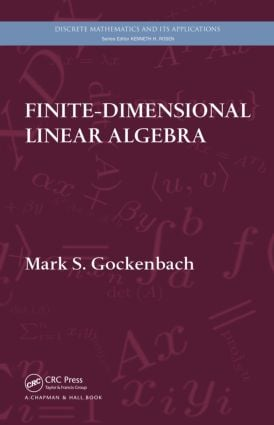# Finite-Dimensional Linear Algebra

## 1st Edition

CRC Press

672 pages | 50 B/W Illus.

Hardback: 9781439815632
pub: 2010-05-06
SAVE ~\$26.00
\$130.00
\$104.00
x
eBook (VitalSource) : 9780429066863
pub: 2010-05-06
from \$65.00

FREE Standard Shipping!

### Description

Linear algebra forms the basis for much of modern mathematics—theoretical, applied, and computational. Finite-Dimensional Linear Algebra provides a solid foundation for the study of advanced mathematics and discusses applications of linear algebra to such diverse areas as combinatorics, differential equations, optimization, and approximation.

The author begins with an overview of the essential themes of the book: linear equations, best approximation, and diagonalization. He then takes students through an axiomatic development of vector spaces, linear operators, eigenvalues, norms, and inner products. In addition to discussing the special properties of symmetric matrices, he covers the Jordan canonical form, an important theoretical tool, and the singular value decomposition, a powerful tool for computation. The final chapters present introductions to numerical linear algebra and analysis in vector spaces, including a brief introduction to functional analysis (infinite-dimensional linear algebra).

Drawing on material from the author’s own course, this textbook gives students a strong theoretical understanding of linear algebra. It offers many illustrations of how linear algebra is used throughout mathematics.

Some Problems Posed on Vector Spaces

Linear equations

Best approximation

Diagonalization

Summary

Fields and Vector Spaces

Fields

Vector spaces

Subspaces

Linear combinations and spanning sets

Linear independence

Basis and dimension

Properties of bases

Polynomial interpolation and the Lagrange basis

Continuous piecewise polynomial functions

Linear Operators

Linear operators

More properties of linear operators

Isomorphic vector spaces

Linear operator equations

Existence and uniqueness of solutions

The fundamental theorem; inverse operators

Gaussian elimination

Newton’s method

Linear ordinary differential equations (ODEs)

Graph theory

Coding theory

Linear programming

Determinants and Eigenvalues

The determinant function

Further properties of the determinant function

Practical computation of det(A)

Eigenvalues and the characteristic polynomial

Diagonalization

Eigenvalues of linear operators

Systems of linear ODEs

Integer programming

The Jordan Canonical Form

Invariant subspaces

Generalized eigenspaces

Nilpotent operators

The Jordan canonical form of a matrix

The matrix exponential

Graphs and eigenvalues

Orthogonality and Best Approximation

Norms and inner products

The adjoint of a linear operator

Orthogonal vectors and bases

The projection theorem

The Gram–Schmidt process

Orthogonal complements

Complex inner product spaces

More on polynomial approximation

The energy inner product and Galerkin’s method

The Helmholtz decomposition

The Spectral Theory of Symmetric Matrices

The spectral theorem for symmetric matrices

The spectral theorem for normal matrices

Optimization and the Hessian matrix

Lagrange multipliers

Spectral methods for differential equations

The Singular Value Decomposition

Introduction to the singular value decomposition (SVD)

The SVD for general matrices

Solving least-squares problems using the SVD

The SVD and linear inverse problems

The Smith normal form of a matrix

Matrix Factorizations and Numerical Linear Algebra

The LU factorization

Partial pivoting

The Cholesky factorization

Matrix norms

The sensitivity of linear systems to errors

Numerical stability

The sensitivity of the least-squares problem

The QR factorization

Eigenvalues and simultaneous iteration

The QR algorithm

Analysis in Vector Spaces

Analysis in Rn

Infinite-dimensional vector spaces

Functional analysis

Weak convergence

Appendix A: The Euclidean Algorithm

Appendix B: Permutations

Appendix C: Polynomials

Appendix D: Summary of Analysis in R

Bibliography

Index

Mark S. Gockenbach is a professor and chair of the Department of Mathematical Sciences at Michigan Technological University.